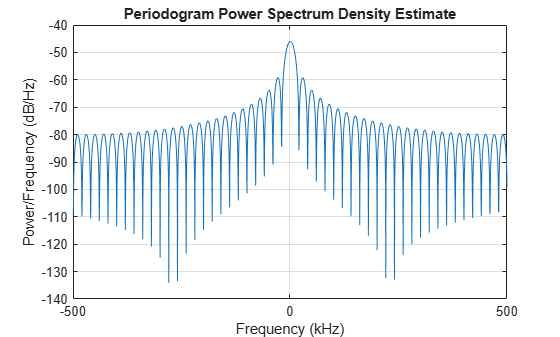Documentation

# phased.StretchProcessor

Stretch processor for linear FM waveform

## Description

The `StretchProcessor` object performs stretch processing on data from a linear FM waveform.

To perform stretch processing:

1. Define and set up your stretch processor. See Construction.

2. Call `step` to perform stretch processing on input data according to the properties of `phased.StretchProcessor`. The behavior of `step` is specific to each object in the toolbox.

### Note

Starting in R2016b, instead of using the `step` method to perform the operation defined by the System object™, you can call the object with arguments, as if it were a function. For example, ```y = step(obj,x)``` and `y = obj(x)` perform equivalent operations.

## Construction

`H = phased.StretchProcessor` creates a stretch processor System object, `H`. The object performs stretch processing on data from a linear FM waveform.

`H = phased.StretchProcessor(Name,Value)` creates a stretch processor object, `H`, with additional options specified by one or more `Name,Value` pair arguments. `Name` is a property name, and `Value` is the corresponding value. `Name` must appear inside single quotes (`''`). You can specify several name-value pair arguments in any order as `Name1,Value1,…,NameN,ValueN`.

## Properties

`SampleRate`

Sample rate

Signal sample rate, specified as a positive scalar. Units are Hertz. The ratio of sample rate to pulse repetition frequency (PRF) must be a positive integer — each pulse must contain an integer number of samples. This property can be specified as single or double precision.

Default: `1e6`

`PulseWidth`

Pulse width

Specify the length of each pulse (in seconds) as a positive scalar. The value must satisfy `PulseWidth <= 1./PRF`. This property can be specified as single or double precision.

Default: `50e-6`

`PRFSource`

Source of pulse repetition values

Source of the PRF values for the stretch processor, specified as `'Property'`, `'Auto'`, or `'Input port'`. When you set this property to `'Property'`, the PRF is determined by the value of the `PRF` property. When you set this property to `'Input port'`, the PRF is determined by an input argument to the `step` method at execution time. When you set this property to `'Auto'`, the PRF is computed from the number of rows in the input signal.

Default: `'Property'`

`PRF`

Pulse repetition frequency

Pulse repetition frequency (PRF) of the received signal, specified as a positive scalar. Units are in Hertz. This property can be specified as single or double precision.

#### Dependencies

To enable this property, set the `PRFSource` property to `'Property'`.

Default: `1`

`SweepSlope`

FM sweep slope

Specify the slope of the linear FM sweeping, in hertz per second, as a scalar.

Default: `2e9`

`SweepInterval`

Location of FM sweep interval

Specify the linear FM sweeping interval using the value `'Positive'` or `'Symmetric'`. If `SweepInterval` is `'Positive'`, the waveform sweeps in the interval between 0 and B, where B is the sweep bandwidth. If `SweepInterval` is `'Symmetric'`, the waveform sweeps in the interval between –B/2 and B/2. This property can be specified as single or double precision.

Default: `'Positive'`

`PropagationSpeed`

Signal propagation speed

Specify the propagation speed of the signal, in meters per second, as a positive scalar. You can specify this property as single or double precision.

Default: Speed of light

`ReferenceRange`

Reference range of stretch processing

Specify the center of ranges of interest, in meters, as a positive scalar. The reference range must be within the unambiguous range of one pulse. This property can be specified as single or double precision. This property is tunable.

Default: `5000`

`RangeSpan`

Span of ranges of interest

Specify the length of the interval for ranges of interest, in meters, as a positive scalar. The range span is centered at the range value specified in the `ReferenceRange` property. This property can be specified as single or double precision.

Default: `500`

## Methods

 step Perform stretch processing for linear FM waveform
Common to All System Objects
`release`

Allow System object property value changes

## Examples

expand all

Use stretch processing to locate a target at a range of 4950 m.

Note: This example runs only in R2016b or later. If you are using an earlier release, replace each call to the function with the equivalent `step` syntax. For example, replace `myObject(x)` with `step(myObject,x)`.

Simulate the signal.

```waveform = phased.LinearFMWaveform; x = waveform(); c = physconst('LightSpeed'); rng = 4950.0; num_samples = round(rng/(c/(2*waveform.SampleRate))); x = circshift(x,num_samples);```

Perform stretch processing.

```stretchproc = getStretchProcessor(waveform,5000,200,c); y = stretchproc(x);```

Plot the spectrum of the resulting signal.

```[Pxx,F] = periodogram(y,[],2048,stretchproc.SampleRate,'centered'); plot(F/1000,10*log10(Pxx)) grid xlabel('Frequency (kHz)') ylabel('Power/Frequency (dB/Hz)') title('Periodogram Power Spectrum Density Estimate')```Detect the range.

```[~,rngidx] = findpeaks(pow2db(Pxx/max(Pxx)),'MinPeakHeight',-5); rngfreq = F(rngidx); rng = stretchfreq2rng(rngfreq,stretchproc.SweepSlope,stretchproc.ReferenceRange,c)```
```rng = 4.9634e+03 ```

expand all

## References

 Richards, M. A. Fundamentals of Radar Signal Processing. New York: McGraw-Hill, 2005.

## See Also

### Topics

#### Hybrid Beamforming for Massive MIMO Phased Array Systems

Download the white paper